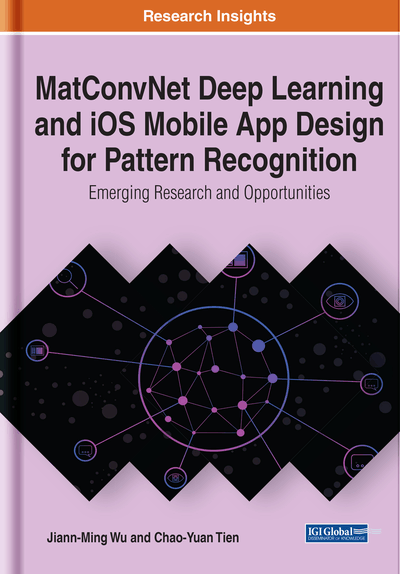# Deep Learning Theory and Software

DOI: 10.4018/978-1-7998-1554-9.ch002
OnDemand:
(Individual Chapters)
Available
\$29.50
No Current Special Offers

## Abstract

In the past decade, deep learning has achieved a significant breakthrough in development. In addition to the emergence of convolution, the most important is self-learning of deep neural networks. By self-learning methods, adaptive weights of kernels and built-in parameters or interconnections are automatically modified such that the error rate is reduced along the learning process, and the recognition rate is improved. Emulating mechanism of the brain, it can have accurate recognition ability after learning. One of the most important self-learning methods is back-propagation (BP). The current BP method is indeed a systematic way of calculating the gradient of the loss with respect to adaptive interconnections. The main core of the gradient descent method addresses on modifying the weights negatively proportional to the determined gradient of the loss function, subsequently reducing the error of the network response in comparison with the standard answer. The basic assumption for this type of the gradient-based self-learning is that the loss function is the first-order differential.
Chapter Preview
Top

## Integrating Data And Cnns Based On Mathematical Deep Learning

Mathematics is the principal core of the development of artificial intelligence. Both machine learning and current deep learning are based on mathematical science. The mathematics is an “engine” for deep learning. Basic mathematics, including calculus, linear algebra and applied linear statistical models, etc., has been applied to deal with identification and classification problems. Deep learning based on the mathematical model induces variant mathematical approaches to discriminant analysis, derivation and calculation of the gradient of the cost function with respect to built-in parameters, the conversion of high-dimensional data to internal representations, and the approximation of the objective function by reducing the error, etc. The importance of mathematics is increasing for the development of AI in the future. The mathematics subjects that developers have studied in the past have great opportunities to enhance recognition accuracy of deep learning or create novel training methods for future AI.

The mathematical software that operates mathematical approaches using computers is critical for deep learning development. Turing machine of Alan Turing in 1936 (Turing, 1937), the Von Neumann architecture in 1945 (Von Neuman, 1993), and the first computer made in accordance with the Von Neumann architecture in the 1950s all tried to expand mathematical knowledge and methods to deal with the huge amount of computations for problem solving. With contributions of Alan Turing and Von Neumann, there is a clear and close connection among mathematics, high performance computing, and artificial intelligence. Today, with the improvement of computing efficiency, we can construct mathematical models on the computer to solve real-world problems which involve extremely high complexity of computations, large-scale data analysis, essential high-dimensional nonlinearity, information uncertainty and data loss. Most of them cannot be easily reduced to an existing mathematical problem. The numerical-analysis software developed by realizing mathematical approaches on high-performance computers helps to develop complex mathematical models. The numerical-analysis software relies on mathematical knowledge and methods that approach problem solving based on solidity of mathematical definitions, theorems and calculations.

The strong connection between mathematics, numerical-analysis software and AI has contributed to the successful development of deep learning. Developers can rely on learned mathematical mapping and use trusted numerical-analysis software to construct deep learning models on high-performance computers to realize the application of AI. Finally, the model of deep learning is used to deal with real-world problems such as image recognition, natural language, and speech analysis in various languages, etc.

## Complete Chapter List

Search this Book:
Reset